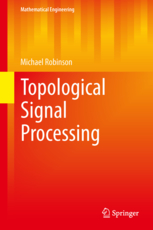# Errata for Topological Signal ProcessingThis page lists errors and corrections for Topological Signal Processing, by Michael Robinson. If you find any errors in the book, please let me know by sending an email to michaelr (at) american (dot) edu. Thanks!

## Chapter 2: Parametrization

• p. 10: The definition of "disjoint union" should be included, or at least referred to an Appendix
• p. 10: There is a slight abuse of notation in the definition of the function $g$ for an adjunction space. Testing for $p \in Y$ is not possible if $p$ is known to be in the disjoint union of $X$ and $Y$ -- the usual definition includes homeomorphic copies of $X$ and $Y$ as subsets of the disjoint union. Since one usually identifies $Y$ with its homeomorphic image via a projection, this is left out of the definition of $g$.
• p. 16: Item (3) in Definition 2.6 has $d,e \in f(c)$ where it should have been $d,e \subseteq f(c)$.
• p. 25: In the statement of Lemma 2.2, it should read $f(U')\subseteq V' \subseteq V$.
• p. 34: Figure 2.26 (right) The two way intersections all say "U1 and U@", but there should also be "U2 and U3" and "U1 and U3".

## Chapter 3: Signals

To spare the reader from excessive verbage, I use the term sheaf to refer to what is usually called a conjunctive cellular presheaf. Because this may cause some confusion, here is a short justification of my choice of terminology. Specifically, a presheaf is a contravariant functor from the category of open sets. A conjunctive presheaf requires that consistent sections can be assembled into sections over larger sets. The usual definition of a sheaf requires that this assembly be unique (for instance Wikipedia). However, signal processing applications often require that consistent sections could be assembled in multiple ways, so the traditional definition of a sheaf is limiting at best.
• p. 44: The restriction map should read $y \pm \frac{1}{2}m_e$ instead of what's written (which is backwards).
• p. 59: In the second line, it says that $\shf{S}(y)$ determines a section over the star of $f^{-1}(y)$. However it should say that $\shf{S}(y)$ determines a section over $f^{-1}(star y)$, the preimage of the star of $y$ through $f$.
• p. 66: The output of the restriction map should be either $(a_1,a_{k+1})$ or $(a_{k+1},a_1)$, not $(a_1,a_k)$ or $(a_k,a_1)$ as written.

## Chapter 4: Detection

• p. 87: The reader might find other examples of forgetful functors an interesting contrast against the concept of a detector.
• p. 94: "But there something more substantial" should read "But there is something more substantial".
• p. 105: The coboundary operator $d$ has $k$ as a superscript where it should read $0$ in three locations: two at the top of the page and one at the bottom.
• p. 109: In the second paragraph, it should have said that the inclusion $Y \to X$ is given the name $f$.
• p. 109: The hypotheses in Theorem 4.4 can be strengthened somewhat. It is not necessary that $H^1(X;A)=0$ in order to obtain an isomorphism; having the connecting map from the snake lemma be the zero map is the sharpest condition.
• p. 119: The restriction maps from the central vertex to the left (input) edges should be projections, but are listed as identity maps.

## Appendix A: Topological spaces

• p.197: The definition of "disjoint union" should be included. An important point is that the usual construction of the disjoint union of $X$ and $Y$ does not include $X$ or $Y$ as subsets. This makes for needlessly complicated exposition, so usually we identify $X$ and $Y$ with homeomorphic copies within the disjoint union through an abuse of notation.